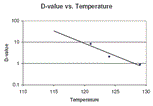# Z-Value Calculation

Source: SGM Biotech, Inc.What is a Z-value?
A Z-value is defined as the number of degrees (Celsius or Fahrenheit) required to change a D-value by one factor of ten. In the practical sense, it is a measure of how susceptible a spore population is to changes in temperature. For example, if the Z-value of a population is 10 degrees, then increasing the sterilization temperature 10 degrees will result in a log reduction of the D-value.

The Z-value can be found by plotting D-values against temperatures on a semilogarithmic scale and adding a line that best fits the data. The absolute value of the reciprocal of the slope of this line will be the Z-value.

Figure 1, D-values at 121, 124, and 129C plotted against Temperature

Since D-values are plotted logarithmically, the line of best fit will be of exponential form and will therefore appear linear on semi-logarithmic paper, as shown above in Figure 1.

Why use a line-of-best-fit?
In a perfect world, the D-value of a particular spore will decrease exponentially as temperature is increased. In reality however, D-values will not decrease perfectly due to natural variations and experimental error. A line-of-best-fit is a statistically correct method of representing the data points.

How do I determine a Z-value graphically?
Calculating a Z-value graphically is relatively easy. Plot at least 3 D-value/temperature pairs on a semi-logarithmic graph and, using your best judgement, draw a straight line through the points that most closely fits the data. From this line, determine the number of degrees required to change the D-value by one factor of 10 (see Figure 2 below).

Figure 2, Calculating a Z-value graphically.

In the above case the Z-value appears to be approximately 8°C.